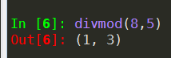• python的数值运算操作符 1.x+y x与y之和 2.x-y x与y之差 3.x*y x与y之积 4.x/y x与y之商 5.x//y x与y之整数商，即不大于x与y之商的最大整数 6.x%y x与y之商的余数，也...python数值运算函数 abs(x) x的绝对值 div...
python的数值运算操作符
1.x+y  x与y之和
2.x-y  x与y之差
3.x*y  x与y之积
4.x/y  x与y之商
5.x//y  x与y之整数商，即不大于x与y之商的最大整数
6.x%y  x与y之商的余数，也称模运算
7.-x  x的负值，即x(-1)*
8.+x  x的本身
9.x**y  x的y次幂，即x^y
python的数值运算函数
abs(x)  x的绝对值
divmod(x,y)  (x//y,x%y)，输入为二元组形式
pow(x,y[,z])  (xy)%z,[……]表示该参数可以省略**
round(x[,ndigits])  对x四舍五入，保留ndigits位小数，round(x)返回四舍五入的整数值
max(x1,x2……,Xn)  x1,x2,……,Xn的最大值，n没有限定
min(x1,x2……,Xn)  x1,x2,……,Xn的最小值，n没有限定
python的数字类型转换函数
int(x)  将x转换为整数，x可以是浮点数或字符串
float(x)  将x转换为浮点数，x可以是整数或字符串
complex(re[,im])  生成一个复数，实部为re，虚部为im，re可以为整数，浮点数或字符串，im可以是整数或浮点数但不能为字符串
简单说说：
s=abs(-3+4j)
#这个的意思是二维坐标系中复数位置到坐标原点的长度
print(s)



展开全文• 1. 数值运算常用函数查询 （1）divmod(a,b)：同时得到a除以b的整除值和余数 （2）int()可以接收浮点数或数字组成的字符串，但无法接收包含小数点或指数的字符串（而float可以）。 （3）python3中，long类型已经...
1. 数值运算常用函数查询

（1）divmod(a,b)：同时得到a除以b的整除值和余数（2）int()可以接收浮点数或数字组成的字符串，但无法接收包含小数点或指数的字符串（而float可以）。

（3）python3中，long类型已经不再存在，而int类型可以存储任意大小的整数，甚至超过64位

（4）round(x, y)，对x进行四舍五入，保留小数点后y位

（5）abs(x) 绝对值； max(x1,x2,...,xn) ， min(x1,x2,...,xn) 最大最小值

（6）complex(x) 将x转换为复数形式

（7）enumerate(x，1)，枚举x中的内容，并为之添加编号（从1开始），x通常为列表类型

展开全文• 数据分析中的常见任务是在大量数字上计算发杂的数学表达式，Python本身提供了所有必须的功能，不同库实现的效率差别很大： 1、 loops = 25000000 from math import * a = range(1,loops) def f(x): return 3 * log...
数据分析中的常见任务是在大量数字上计算复杂的数学表达式，Python本身提供了所有必须的功能，不同库实现的效率差别很大：
1、
loops = 25000000
from math import *
a = range(1,loops)
def f(x):
return 3 * log(x) + cos(x) ** 2
%timeit r = [f(x) for x in a]

18.9 s ± 221 ms per loop (mean ± std. dev. of 7 runs, 1 loop each)
2、使用Numpy可以实现大幅优化
import numpy as np
loops = 25000000
a = np.arange(1,loops)

%timeit r = 3 * np.log(a) + np.cos(a) ** 2

1.12 s ± 10.2 ms per loop (mean ± std. dev. of 7 runs, 1 loop each)
3、甚至有一个库是专门用于此类任务的，这个库称作numexpr，得名于“数值表达式”(Numerical Expressions)。它编译表达式，改善NumPyl通用功能的性能，例如，在执行期间避免数组在内存中复制:
import numexpr as ne
ne.set_num_threads(1)
f = '3 * log(a) + cos(a) ** 2'
%timeit r = ne.evaluate(f)

476 ms ± 7.11 ms per loop (mean ± std. dev. of 7 runs, 1 loop each)
4、numexpr还内建并行执行单独运算的功能。使用4线程可进一步降低运行时间
ne.set_num_threads(4)
f = '3 * log(a) + cos(a) ** 2'
%timeit r = ne.evaluate(f)

218 ms ± 3.85 ms per loop (mean ± std. dev. of 7 runs, 1 loop each)


展开全文• #pow(x,y,z)幂运算，x的y次方，z的运算是(x**y % z) >>> pow(2,3) 8 >>> 2**3 8 >>> pow(2,3,4) # 8 % 4 = 2......0,所以余除是0 0 #max()和min(),分别是取最大值和最小值，如果不是同类型比较就会报错 >>> max...
#abs(x)绝对值的x

>>> abs(-30)
30
>>> abs(-10)
10
>>> abs(-20)
20
>>> abs(40)
40
>>> abs(-10+30e3j)
30000.00166666662
>>> 10e2                        #10e2等于10×10的2次方
1000.0
>>> abs((-10+30e3j).imag)       #imag取虚数部分
30000.0
>>> abs((-10+30e3j).real)       #real取实数部分
10.0

#divmod（x,y）返回元组类型，输出（x // y,x % y）
>>> divmod(10,10)
(1, 0)
>>> a,b = divmod(10,10)
>>> a                    #10 // 10 = 1,所以 a == 1
1
>>> b                    #10 % 10 = 1 ......0 ，所以 b == 0
0

#pow(x,y,z)幂运算，x的y次方，z的运算是(x**y % z)
>>> pow(2,3)
8
>>> 2**3
8
>>> pow(2,3,4)       # 8 % 4 = 2......0,所以余除是0
0

#max()和min(),分别是取最大值和最小值，如果不是同类型比较就会报错
>>> max(-1,0,1,2,3,4,'5')
Traceback (most recent call last):
File "<pyshell#0>", line 1, in <module>
max(-1,0,1,2,3,4,'5')
TypeError: '>' not supported between instances of 'str' and 'int'
>>> max(-1,0,1,2,3,4,5)
5
>>> min(-1,0,1,2,3,4,5)
-1


展开全文• Python常用的内置数值运算函数: 1、abs(x):求x的绝对值 2、divmod(x,y):(x//y,x%y),输出为二元组形式 3、pow(x,y[,z]):(x**y)%z,[…]表示参数可省,即pow(x,y),同x**y 4、round(x[ndigits]对x四舍五入,保留ndigits位...
• 转贴：来源: http://hi.baidu.com/leechl转载请注明来源: http://hi.baidu.com/leechlPython有5个内建的数值运算函数, 虽然很简单的函数, 还是从Python核心编程哪里摘录几段做一下笔记吧.abs()返回给定参数的...
• 运算符⽤于执⾏程序代码运算，会针对⼀个以上操作数项⽬来进⾏运算。例 如：2+3，其操作数是2和3，⽽运算符则是“+” 1.2运算符的分类 算术运算符 赋值运算符 ⽐较运算符（关系运算符） 逻辑运算符 条件运算符（三元...
• 一、数字类型 （一）整数类型int（integer），与数学中的整数概念一致 特点：可正可负，没有取值范围 ...各种进制相互转换函数 十进制int(), 八进制oct(), 十六进制hex() 注：单单写一个阿拉伯数字，是十进制整数
• 1、数值运算函数 abs(x) #取x的绝对值 divmod(x,y) #等于(x//y,x%y)，先取整再取余 pow(x,y,z) #等于(x**y)%z，先取x的y次方，再对z取余 max() #取最大值 min() #取最小值 2、字符串处理函数 len(x) ...字符串
• 数值运算操作符 操作符及使用 描述 x+y 加，x与y之和 x-y 减，x与y之差 x*y 乘，x与y之积 x/y 除，x与y之商 x//y 整除，x与y整数商 +x x本身 -y y的复数 x%y 余数 x**y 幂运算 示例： ...
• 浮点数间运算及比较用round()函数辅助 科学记数法的表示方式：<a>e<b>表示a*10的b次方 复数 z=4+5j 实部：用z.real获得实部 虚部：用z.imag获得虚部 操作符 +（加）、-(减)、*（乘）、/（除）结果是......

# python数值运算函数python 订阅